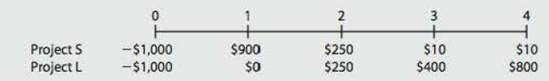Chapter 11, Problem 12PFundamentals of Financial Manageme...

14th Edition
Eugene F. Brigham + 1 other
ISBN: 9781285867977

Solutions

Chapter
SectionFundamentals of Financial Manageme...

14th Edition
Eugene F. Brigham + 1 other
ISBN: 9781285867977
Textbook Problem

IRR AND NPV A company is analyzing two mutually exclusive projects, S and L, with the following cash flows:The company’s WACC is 10%. What is the IRR of the better project? (Hint: The better project may or may not be the one with the higher IRR.)

Summary Introduction

To calculate: The IRR of the better project.

Net Present Value (NPV):

It is a method under capital budgeting, which includes the calculation of net present value of the project in which company is investing. The calculation is done by calculating the difference between the value of cash inflow and value of cash outflow after taking into consideration the discounted rate.

Internal Rate of Return (IRR):

It refers to the rate of return that is computed by the company to make a decision of selection of a project for investment. This rate provides the basis for selection of projects with lower cost of capital and rejection of project with higher cost of capital.

Explanation

The calculation of NPV and IRR of project S on spreadsheet:

Table (1)

The NPV of the project S is \$39.13 and IRR is 13%.

The calculation of NPV and IRR of project L on spreadsheet is:

Still sussing out bartleby?

Check out a sample textbook solution.

See a sample solution

The Solution to Your Study Problems

Bartleby provides explanations to thousands of textbook problems written by our experts, many with advanced degrees!

Get Started

Find more solutions based on key concepts